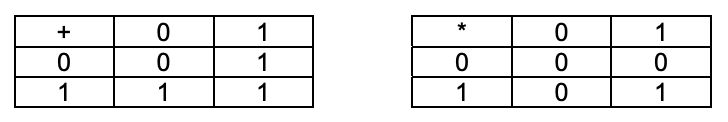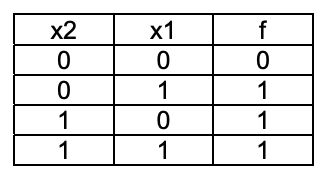시간 제한 메모리 제한 제출 정답 맞은 사람 정답 비율
1 초 128 MB 0 0 0 0.000%

## 문제

Each mapping f of the set {0,1}n of n-dimensional binary vectors to {0,1} is called Boolean function of n variables and denoted by f(xn, xn-1, …, x1). For cryptography some properties of the Boolean functions are interesting. Let denote by B(n,k) the set of n-dimensional binary vectors that have k 1’s. The task is for given Boolean function f to find the number of vectors (bn, bn-1, …, b1) from B(n,k) such that f(bn, bn-1, …, b1)=1.

The Boolean function will be given by its (unique) polynomial modulo 2. In these polynomials the operations addition and multiplication modulo 2 are used, defined as shown in the tables of Fig. 1. In the polynomial of a function any product of m variables xi1 xi2 K xim could participate or not participate. So the general form of the polynomial for n variables is:

a0 + a1x1 + a2x2 + a3x2x1 + a4x3 + a5x3x1 + a6x3x2 + a7x3x2x1 + . . . + aNxnxn-1…x1

where all coefficients aj, j=0,1,…,N=2n -1, are 0’s or 1’s and if the coefficient is equal to 0 we will omit the corresponding product and if it is equal to 1 we just will omit the coefficient. For example, the polynomial of the Boolean function disjunction of 2 variables given on Fig. 2 is 0 + 1.x1 + 1.x2 + 1.x2x1 = x1 + x2 + x2x1.Fig. 1Fig. 2

## 입력

Your program has to be ready to solve more than one test case. The first line of the input file will contains only the number T of the test cases. Each of the following T lines will describe one function – first the numbers n and k separated by single space (1≤n≤18,0≤k≤n) and then, separated by one more space a string of 2n 0’s and 1’s that are the coefficients of the corresponding polynomial, ordered as in the general form of the polynomial given above.

## 출력

The output file have to contain T lines with a single number each – the number of vectors found by your program.

## 예제 입력 1

3
2 1 0111
4 2 1000000000000000
5 3 00000000000000000000000000000001


## 예제 출력 1

2
6
0